Courses

# NCERT Solutions Chapter 6 - Integers, Maths, Class 6 | EduRev Notes

## Class 6 : NCERT Solutions Chapter 6 - Integers, Maths, Class 6 | EduRev Notes

The document NCERT Solutions Chapter 6 - Integers, Maths, Class 6 | EduRev Notes is a part of the Class 6 Course Mathematics (Maths) Class 6.
All you need of Class 6 at this link: Class 6

Exercise 6.1

Question 1

Write opposites of the following:

(a) Increase in weight

(b) 30 km north

(c) 326 BC

(d) Loss of Rs 700

(e) 100 m above sea level

Answer 1: (a) Decrease in weight

(b) 30 km south

(d) Profit of Rs. 700

(e) 100 m below sea level

Question 2

Represent the following numbers as integers with appropriate signs.

(a) An aeroplane is flying at a height two thousand metre above the ground.

(b) A submarine is moving at a depth, eight hundred metre below the sea level.

(c) A deposit of rupees two hundred.

(d) Withdrawal of rupees seven hundred.

(a) (+) 2000 meters

(b) (-) 800 meters

(c) (+) 200 Rupees

(d) (-) 700 Rupees

Question 3

Represent the following numbers on a number line:

(a) + 5

(b) -10

(c) + 8

(d) -1

(e) -6

(a)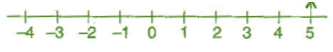(b)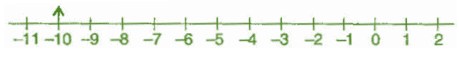(c)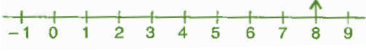(d)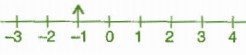(e)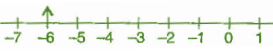Question 4

Adjacent figure is a vertical number line, representing integers. Observe it and locate the following points:

(a) If point D is + 8, then which point is -8?

(b) Is point G a negative integer or a positive integer?

(c) Write integers for points B and E.

(d) Which point marked on this number line has the least value?

(e) Arrange all the points in decreasing order of value.

(b) Negative

(c) B = (+) 4; E = (-) 10

(d) E

(e) D, C, B, A, O, H, G, F, E

Question 5

Following is the list of temperatures of five places in India on a particular day of the year.

 Place Temperature Siachin 10°C below 0°C ........... Shirnla 2°C below 0°C............ Ahmedabad 30°C above 0°C............. Delhi 20°C above 0°C............. Srinagar 5°C below 0°C...........

(a) Write the temperatures of these places in the form of integers in the blank column.

(b) Following is the number line representing the temperature in degree Celsius.

Plot the name of the city against its temperature.

(c) Which is the coolest place?

(d) Write the names of the places where temperatures are above 10°C.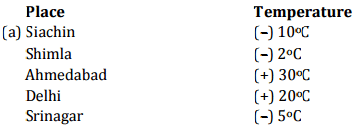(b) Number line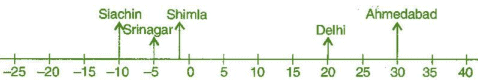(c) Siachin

Question 6

In each of the following pairs, which number is to the right of the other on the number line?

(a) 2, 9

(b) -3, -8

(c) 0, -1

(d) -11, 10

(e) -6, 6

(f) 1, -100

(a) 9 is right to 2

(b) -3 is right to -8

(c) 0 is right to -1

(d) 10 is right to -11

(e) 6 is right to -6

(f) 1 is right to -100

Question 7

Write all the integers between the given pairs (write them in the increasing order.)

(a) 0 and -7

(b) -4 and 4

(c) -8 and -15

(d) -30 and -23

(a) -6, -5, -4, -3, -2, -1

(b) -3, -2, -1, 0, 1, 2, 3

(c) -14, -13, -12, -11, -10, -9

(d) -29, -28, -27, -26, -25, -24

Question 8

(a) Write four negative integers greater than -20.

(b) Write four negative integers less than -10.

(a) -19, -18, -17, -16

(b) -11, -12, -13, -14

Question 9

For the following statements, write True (T) or False (F). If the statement is false, correct the statement.

(a) -8 is to the right of -10 on a number line.

(b) -100 is to the right of -50 on a number line.

(c) Smallest negative integer is -1

(d) -26 is greater than -25.

(a) True

(b) False

(c) False

(d) False

Question 10

Draw a number line and answer the following:

(a) Which number will we reach if we move 4 numbers to the right of -2.

(b) Which number will we reach if we move 5 numbers to the left of 1.

(c) If we are at -8 on the number line, in which direction should we move to reach -13?

(d) If we are at -6 on the number line, in which direction should we move to reach -1?

(a)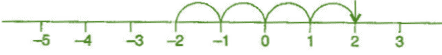(b)(c) On left side

(d) On right side

Exercise 6.2

Question 1

Using the number line write the integer which is:

(a) 3 more than 5

(b) 5 more than -5

(c) 6 less than 2

(d) 3 less than -2

(a) 8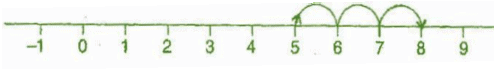(b)  0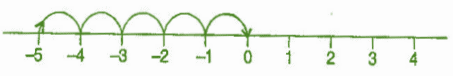(c) -4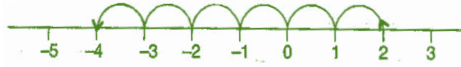(d) -5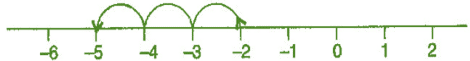Question 2

Use number line and add the following integers:

(a) 9 + (-6)

(b) 5 + (-11)

(c) (-1) + (-7)

(d) (-5) + 10

(e) (-1) + (-2) + (-3)

(f) (-2) + 8 + (-4)

(a) 9 + (-6) = 3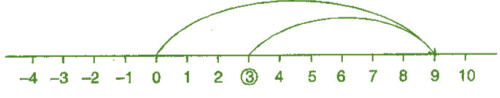(b) 5 + (-11) = -6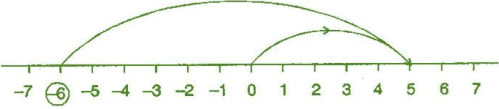(c) (-1) + (-7) = -8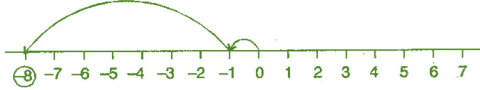|

(d) (-5) + 10 = 5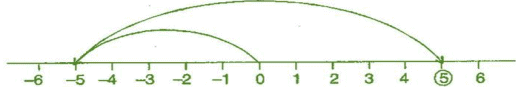(e) (-1) + (-2) + (-3) = -6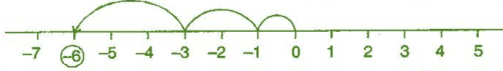(f) (-2) + 8 +(-4) = 2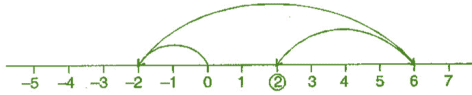Question 3

(a) 11 + (-7)

(b) (-13) + (+18)

(c) (-10) + (+19)

(d) (-250) + (+ 150)

(e) (-380) + (-270)

(f) (-217) + (-100)

Answer 3: (a) 11 + (-7) = 11 - 7 = 4

(b) (-13) + 18 = 5

(c) (-10) + (+19) = -10 + 19 = 9

(d) (-250) + (+150) = -250 + 150 = -100

(e) (-380) + (-270) = -380 - 270 = -650

(f) (-217) + (-100) = -217 - 100 = -317

Question 4

(a) 137 and -354

(b) -52 and 52

(c) -312, 39 and 192

(d) -50, -200 and 300

(a) 137 + (-354) = 137 - 354 = -217

(b) (-52) + 52 = 0

(c) (-312) + 39 + 192 = -312 + 231 = -81

(d) (-50) + (-200) + 300 = -50 - 200 + 300 = -250 + 300 = 50

Question 5

Find the sum:

(a) (-7) + (-9) + 4 + 16

(b) (37) + (-2) + (-65) + (-8)

(a) (-7) + (-9) + 4 + 16

= -7 - 9 + 4 + 16

= -16 + 20

= 4

(b) 37 + (-2) + (-65) + (-8)

= 37 - 2 - 65 - 8

= 37 - 75

= -38

Exercise 6.3

Question 1: Subtract:

(a) 35 - (20)

(b) 72 - (90)

(c) (-15) - (-18)

(d) (-20) - (13)

(e) 23 - (-12)

(f) (-32) - (-40)

Answer 1: (a) 35 - 20 = 15

(b) 72 - 90 = -18

(c) (-15) - (-18)

= -15 + 18

= 3

(d) -20 - (13)

= -20 - 13

= -33

(e) 23 - (-12)

= 23 + 12

= 35

(f) (-32) - (-40)

= -32 + 40

= 8

Question 2: Fill in the blanks with >, < or = sign:

(a) (-3) + (-6) _______________ (-3) - (-6)

(b)(-21) - (-10) _______________ (-31) + (-11)

(c) 45 - (-11) _______________ 57 + (-4)

(d)(-25) - (-42) _______________ (-42) - (-25)

(a) (-3) + (-6)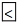(-3) - (-6)

(b) (-21) - (-10)(-31) + (-11)

(c) 45 - (-11)57 + (-4)

(d) (-25) - (-42)(-42) - (-25)

Question 3:

Fill in the blanks:

(a) (-8) + __________ = 0

(b) 13 + __________ = 0

(c) 12 + (-12) = __________

(d)(-4) + __________ = -12

(e) __________ - 15 = -10

(a) (-8) + 8 = 0

(b) 13 + (-13) = 0

(c) 12 + (-12) = 0

(d) (-4) + (-8) = -12

(e) 5 - 15 = -10

Question 4: Find:

(a) (-7) - 8 - (-25)

(b)(-13) + 32 - 8 - 1

(c) (-7) + (-8) + (-90)

(d) 50 - (-40) - (-2)

(a) (-7) - 8 - (-25)

= -7 - 8 + 25

= -15 + 25

= 10

(b) (-13) + 32 - 8 - 1

= -13 + 32 - 8 - 1

= 32 - 22

= 10

(c) (-7) + (-8) + (-90)

= -7 - 8 - 90

= -105

(d) 50 - (-40) - (-2)

= 50 + 40 + 2

= 92

Offer running on EduRev: Apply code STAYHOME200 to get INR 200 off on our premium plan EduRev Infinity!

,

,

,

,

,

,

,

,

,

,

,

,

,

,

,

,

,

,

,

,

,

,

,

,

,

,

,

;